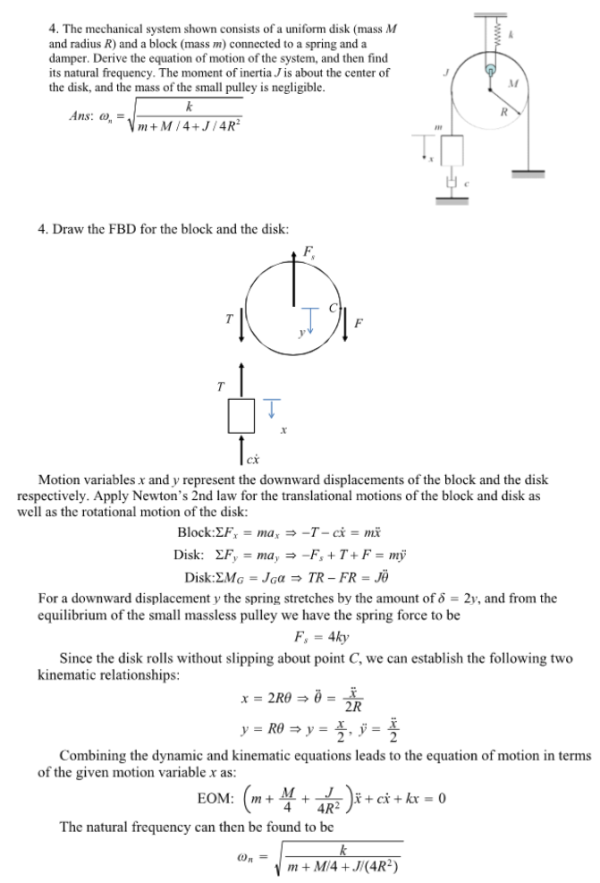The mechanical system shown consists of a uniform disk (mass M and radius R) and a block (mass m) connected to a spring and a damper. Derive the equation of motion of the system, and then find its natural frequency. The moment of inertia J is about the center of the disk, and the mass of the small pulley is negligible.The mechanical system shown consists of a uniform disk (mass M and radius R) and a block (mass m) connected to a spring and a damper. Derive the equation of motion of the system, and then find its natural frequency. The moment of inertia J is about the center of the disk, and the mass of the small pulley is negligible.

System Dynamics Page 2 dynamics dynamics dynamics dynamics dynamics dynamics dynamics System dynamics Page 3